• ## 基于深度学习的变化检测算法实现

千次阅读 热门讨论 2019-06-12 23:04:37
我是研究生期间研究主要研究SAR影像的变化检测，这是一段简单的基于深度学习变化检测方法，以CNN实现。 后续我会把整个代码的思路写上，由于这个是自己写的代码，如果有哪些地方写的不太好请大家见谅，如果有不懂...
我是研究生期间研究主要研究SAR影像的变化检测，这是一段简单的基于深度学习的变化检测方法，以CNN实现。
首先说下基于深度学习的变化检测任务的思路：
制作训练样本 -> 训练模型  ->  使用训练的模型遍历图片中每个像元得出结果
1 筛选训练样本
第一步，通过两幅影像生成差异图。
第二部，使用一个简单的方法对差异图进行分割，生成一幅较为粗略的二值图 。
第三部，选取变化样本和未变化样本，选择方式一般有两种：

1 从差异图中选择%T像素值最大和最小的像元，每个像元取其邻域分别作为变化样本和未变化样本；
2 从生成的粗略二值图中随机选择一部分像元，每个像元根据其类别取其邻域分别作为变化样本和未变化样本；

2 训练模型
将制作好的变化样本和未变化样本输入模型中进行训练，模型的选择通常有:

受限玻尔兹曼机(RBM)，使用最多
降噪自编码器（DAE）
卷积神经网络（CNN）

3 得出变化图
直接使用训练的模型遍历图片中每个像元的邻域对每个像元进行二分类即可得出检测结果。
虽然是走的监督学习的路线，但其并没有用到人工标注的gt，本质上该变化检测任务是一种非监督方法。
从深度学习的角度上讲，它属于图像分类的领域，相当于对每个像素的邻域（3×3，5×5，7×7，9×9）进行二分类。
实例代码
代码是自己写的，里面有很多地方可以完善，后续会慢慢完善，筛选样本部分耗时特别大，这里大家可自行完善一下（排序方式改一改就能快很多），或改成随机选择训练样本，注意在这个代码不更改的情况下最好只使用500×500左右的图像进行检测，太大的话会跑不动。
输入两幅影像：
import tensorflow as tf
import numpy as np
import cv2 as cv
# 读取影像
path = 'E:/data changing2/'

img1 = cv.copyMakeBorder(img1, 2, 2, 2, 2, cv.BORDER_REFLECT)   #给影像填充边界
img2 = cv.copyMakeBorder(img2, 2, 2, 2, 2, cv.BORDER_REFLECT)

img_row = img1.shape   # 行
img_columns = img1.shape     # 列

img1 = np.asarray(img1, np.float32)
img2 = np.asarray(img2, np.float32)

生成差异图和差异二值图：
img_change = []
temp = np.longfloat(0)
for i in range(img_row):
for j in range(img_columns):
temp = np.square(img1[i][j]-img2[i][j]) + np.square(img1[i][j]-img2[i][j])\
+ np.square(img1[i][j]-img2[i][j])
temp = np.sqrt(temp)
img_change.append(temp)

# img_change = np.asarray(img_change, np.float32)
# max_ = img_change.max()
# min_ = img_change.min()
max_ = max(img_change)
min_ = min(img_change)
print('max = ', max_, 'min = ', min_)
for i in range(len(img_change)):
img_change[i] = (img_change[i]-min_)/(max_ - min_)*255

# 生成差异图和差异二值图
img_gray = [[0 for col in range(img_columns)] for row in range(img_row)]
img_gray = np.asarray(img_gray, np.float32)

k = 0
for i in range(img_row):
for j in range(img_columns):
img_gray[i][j] = img_change[k]
k += 1

img_gray01 = cv.imread(path + 'FCM.bmp', 0)   # 使用一个比较粗的结果来筛选纯净样本
img_gray01 = cv.copyMakeBorder(img_gray01, 2, 2, 2, 2, cv.BORDER_REFLECT)
img_gray = np.asarray(img_gray, np.uint8)
img_gray01 = np.asarray(img_gray01, np.uint8)

# io.imsave(path + 'chayitu.bmp', img_gray)
# io.imsave(path + '2zhitu.bmp', img_gray01)
print("差异图，基础二值图生成完毕")

制作训练样本：
这里采用简单选择排序，邻域大小选择为7*7.
# 1. 给 img_change 排序(只排序（T%*2）以加快程序运行速度)   2. 选出(T% * 2)的样本作为训练数据集并生成相应的图像
# 1

# T 阈值
T = 0.02
T_num = int(len(img_change)*T)
print("changdu:", len(img_change))
print('T:', T_num)
#  将img_change的值赋给img_change_sort(切记不能用 = !!!! 这个会共享数据)
img_change_sort = []
for i in range(len(img_change)):
img_change_sort.append(img_change[i])
label_change = []
for i in range(len(img_change)):
label_change.append(i)

for i in range(T_num):
temp_max = img_change_sort[i]
img_label_1 = i
for j in range(i, len(img_change)):
if img_change_sort[j] > temp_max:
temp_max = img_change_sort[j]
img_label_1 = j
img_change_sort[img_label_1] = img_change_sort[i]
img_change_sort[i] = temp_max

label_change[i] = label_change[img_label_1]
label_change[img_label_1] = i

for i in range(T_num):
temp_min = img_change_sort[len(img_change_sort)-i-1]
img_label_0 = len(img_change_sort) - i - 1
for j in range(len(img_change)-i):
if img_change_sort[j] < temp_min:
temp_min = img_change_sort[j]
img_label_0 = j
img_change_sort[img_label_0] = img_change_sort[len(img_change_sort)-i-1]
img_change_sort[len(img_change_sort)-i-1] = temp_min

label_change[len(img_change_sort)-i-1] = label_change[img_label_0]
label_change[img_label_0] = len(img_change_sort) - i - 1

# 2
data = []
label = []
data1 = []
data0 = []
img_1 = [[0 for col1 in range(img_columns)] for row1 in range(img_row)]
ratio = 0.88
data_1_count = 0
data_0_count = 0
for i in range(img_row):
for j in range(img_columns):
img_1[i][j] = 125
for i in range(T_num):
region = []
row_1 = int(label_change[i]/img_columns)
column_1 = label_change[i] % img_columns
if row_1 < 2 or column_1 < 2 or row_1 >= (img_row-2) or column_1 >= (img_columns - 2):
continue
else:
count = 0
for k in range(row_1 - 2, row_1 + 3):
for l in range(column_1 - 2, column_1 + 3):
if img_gray01[k][l] == 255:
count += 1
if count / 25 >= ratio:
# if count / 49 >= 0.5:
for k in range(row_1 - 2, row_1 + 3):
for l in range(column_1 - 2, column_1 + 3):
for m in range(3):
region.append(img1[k][l][m])
for n in range(3):
region.append(img2[k][l][n])
data1.append(region)
data_1_count += 1
img_1[row_1][column_1] = 255
data1 = np.asarray(data1, np.float32)

for i in range(T_num):
row_0 = int(label_change[len(img_change)-i-1] / img_columns)
column_0 = label_change[len(img_change)-i-1] % img_columns
region = []
if row_0 < 2 or column_0 < 2 or row_0 >= (img_row-2) or column_0 >= (img_columns - 2):
continue
else:
count = 0
for k in range(row_0 - 2, row_0 + 3):
for l in range(column_0 - 2, column_0 + 3):
if img_gray01[k][l] == 0:
count += 1
if count / 25 >= ratio:
# if count / 25 >= 0.6:
for k in range(row_0 - 2, row_0 + 3):
for l in range(column_0 - 2, column_0 + 3):
for m in range(3):
region.append(img1[k][l][m])
for n in range(3):
region.append(img2[k][l][n])
data0.append(region)
# label.append(0)
data_0_count += 1
img_1[row_0][column_0] = 0
img_1 = np.asarray(img_1, np.int64)
cv.imwrite(path + 'xunlianyangben.bmp', img_1)
data0 = np.asarray(data0, np.float32)

c = min(data1.shape, data0.shape)
for i in range(c):
data.append(data1[i])
label.append(1)
data.append(data0[i])
label.append(0)

data = np.asarray(data, np.float32)
label = np.asarray(label, np.int32)
print(data_1_count, data_0_count)
print('data.shape:', np.shape(data))

# 打乱样本顺序
num_example = data.shape
arr = np.arange(num_example)
np.random.shuffle(arr)
data = data[arr]
label = label[arr]

制作测试样本：
测试样本就是直接遍历整幅影像，它经过模型后输出的便是变化检测结果， 这里发现如果一个列表太大的时候会出现问题，就分成两个数组分别保存了
# 制作测试样本
data_test = []
label_test = []
for i in range(202):
for j in range(img_columns):
if i < 2 or j < 2 or i >= (img_row-2) or j >= (img_columns - 2):
continue
else:
region = []
for k in range(i - 2, i + 3):
for l in range(j - 2, j + 3):
for m in range(3):
region.append(img1[k][l][m])
for n in range(3):
region.append(img2[k][l][n])
data_test.append(region)
label_test.append(img_gray01[i][j]/255)
data_test = np.asarray(data_test, np.float32)
label_test = np.asarray(label_test, np.int32)

data_test1 = []
label_test1 = []
for i in range(202, img_row):
for j in range(img_columns):
if i < 2 or j < 2 or i >= (img_row-2) or j >= (img_columns - 2):
continue
else:
region = []
for k in range(i - 2, i + 3):
for l in range(j - 2, j + 3):
for m in range(3):
region.append(img1[k][l][m])
for n in range(3):
region.append(img2[k][l][n])
data_test1.append(region)
label_test1.append(img_gray01[i][j]/255)
data_test1 = np.asarray(data_test1, np.float32)
label_test1 = np.asarray(label_test1, np.int32)

创建并训练模型：
data = np.reshape(data, [len(data), 5, 5, 6])
data_test = np.reshape(data_test, [len(data_test), 5, 5, 6])
data_test1 = np.reshape(data_test1, [len(data_test1), 5, 5, 6])
# -----------------构建网络----------------------
# 占位符
x = tf.placeholder(tf.float32, shape=[None, 5, 5, 6], name='x')
y_ = tf.placeholder(tf.int32, shape=[None, ], name='y_')

# 第一个卷积层（5——>5)
conv1 = tf.layers.conv2d(
inputs=x,
filters=32,
kernel_size=[3, 3],
activation=tf.nn.relu,
kernel_initializer=tf.truncated_normal_initializer(stddev=0.01))

# 第二个卷积层(5->5)
conv2 = tf.layers.conv2d(
inputs=conv1,
filters=32,
kernel_size=[3, 3],
activation=tf.nn.relu,
kernel_initializer=tf.truncated_normal_initializer(stddev=0.01))
pool1 = tf.layers.max_pooling2d(inputs=conv2, pool_size=[2, 2], strides=1)

re1 = tf.reshape(pool1, [-1, 4 * 4 * 32])

# 全连接层
logits = tf.layers.dense(inputs=re1,
units=2,
activation=tf.nn.softmax,
kernel_initializer=tf.truncated_normal_initializer(stddev=0.01),
kernel_regularizer=tf.contrib.layers.l2_regularizer(0.003))

loss = tf.losses.sparse_softmax_cross_entropy(labels=y_, logits=logits)
correct_prediction = tf.equal(tf.cast(tf.argmax(logits, 1), tf.int32), y_)
acc = tf.reduce_mean(tf.cast(correct_prediction, tf.float32))

# 定义一个函数，按批次取数据
def minibatches(inputs=None, targets=None, batch_size=None, shuffle=False):
assert len(inputs) == len(targets)
if shuffle:
indices = np.arange(len(inputs))
np.random.shuffle(indices)
for start_idx in range(0, len(inputs) - batch_size + 1, batch_size):
if shuffle:
excerpt = indices[start_idx:start_idx + batch_size]
else:
excerpt = slice(start_idx, start_idx + batch_size)
yield inputs[excerpt], targets[excerpt]

# 训练CNN模型，可将n_epoch设置更大一些

n_epoch = 20
batch_size = 128
sess = tf.InteractiveSession()
sess.run(tf.global_variables_initializer())
for epoch in range(n_epoch):
start_time = time.time()

# training
train_loss, train_acc, n_batch = 0, 0, 0
for x_train_a, y_train_a in minibatches(data, label, batch_size, shuffle=True):
_, err, ac = sess.run([train_op, loss, acc], feed_dict={x: x_train_a, y_: y_train_a})
train_loss += err
train_acc += ac
n_batch += 1
print("   train loss: %f" % (train_loss / n_batch))
# print("   train acc: %f" % (train_acc / n_batch))

将测试样本输入训练过模型得出最终结果：
# 用训练好的模型得出结果
final_img = []
final = sess.run(logits, feed_dict={x:  data_test, y_: label_test})
for i in range(len(data_test)):
if final[i] >= final[i]:
finals = 0
else:
finals = 255
final_img.append(finals)
print(len(final_img))
k = 0

img_final2 = [[125 for i in range(img_columns-4)] for j in range(img_row-4)]
for i in range(200):
for j in range(img_columns-4):
img_final2[i][j] = final_img[k]
k += 1

final_img1 = []
final1 = sess.run(logits, feed_dict={x:  data_test1, y_: label_test1})
for i in range(len(data_test1)):
if final1[i] >= final1[i]:
finals = 0
else:
finals = 255
final_img1.append(finals)
print(len(final_img1))
k = 0
for i in range(img_row-4-200):
for j in range(img_columns-4):
img_final2[i+200][j] = final_img1[k]
k += 1

img_final2 = np.asarray(img_final2, np.uint8)
cv.imwrite(path + 'final/2zhitu_final_not_use_CNN_5_new.bmp', img_final2)
sess.close()

完整代码: https://blog.csdn.net/weixin_39853245/article/details/97670214


展开全文SAR 卷积神经网络
• 详解见： ...代码如下： import tensorflow as tf import numpy as np import time import cv2 as cv # 读取影像 path = 'E:/data changing2/' img1 = cv.imread(pa...
详解见： https://blog.csdn.net/weixin_39853245/article/details/91650607
代码如下：
import tensorflow as tf
import numpy as np
import time
import cv2 as cv
# 读取影像
path = 'E:/data changing2/'

img1 = cv.copyMakeBorder(img1, 2, 2, 2, 2, cv.BORDER_REFLECT)
img2 = cv.copyMakeBorder(img2, 2, 2, 2, 2, cv.BORDER_REFLECT)

img_row = img1.shape   # 行
img_columns = img1.shape     # 列

img1 = np.asarray(img1, np.float32)
img2 = np.asarray(img2, np.float32)

# 生成差异图
img_change = []
temp = np.longfloat(0)
for i in range(img_row):
for j in range(img_columns):
temp = np.square(img1[i][j]-img2[i][j]) + np.square(img1[i][j]-img2[i][j])\
+ np.square(img1[i][j]-img2[i][j])
temp = np.sqrt(temp)
img_change.append(temp)

# img_change = np.asarray(img_change, np.float32)
# max_ = img_change.max()
# min_ = img_change.min()
max_ = max(img_change)
min_ = min(img_change)
print('max = ', max_, 'min = ', min_)
for i in range(len(img_change)):
img_change[i] = (img_change[i]-min_)/(max_ - min_)*255

# 生成差异图和差异二值图
img_gray = [[0 for col in range(img_columns)] for row in range(img_row)]
img_gray = np.asarray(img_gray, np.float32)

k = 0
for i in range(img_row):
for j in range(img_columns):
img_gray[i][j] = img_change[k]
k += 1

img_gray01 = cv.imread(path + 'FCM.bmp', 0)   # 使用一个比较粗的结果来筛选纯净样本
img_gray01 = cv.copyMakeBorder(img_gray01, 2, 2, 2, 2, cv.BORDER_REFLECT)
img_gray = np.asarray(img_gray, np.uint8)
img_gray01 = np.asarray(img_gray01, np.uint8)

# io.imsave(path + 'chayitu.bmp', img_gray)
# io.imsave(path + '2zhitu.bmp', img_gray01)
print("差异图，基础二值图生成完毕")

# 1. 给 img_change 排序(只排序（T%*2）以加快程序运行速度)   2. 选出(T% * 2)的样本作为训练数据集并生成相应的图像
# 1

# T 阈值
T = 0.02
T_num = int(len(img_change)*T)
print("changdu:", len(img_change))
print('T:', T_num)
#  将img_change的值赋给img_change_sort(切记不能用 = !!!! 这个会共享数据)
img_change_sort = []
for i in range(len(img_change)):
img_change_sort.append(img_change[i])
label_change = []
for i in range(len(img_change)):
label_change.append(i)

for i in range(T_num):
temp_max = img_change_sort[i]
img_label_1 = i
for j in range(i, len(img_change)):
if img_change_sort[j] > temp_max:
temp_max = img_change_sort[j]
img_label_1 = j
img_change_sort[img_label_1] = img_change_sort[i]
img_change_sort[i] = temp_max

label_change[i] = label_change[img_label_1]
label_change[img_label_1] = i

for i in range(T_num):
temp_min = img_change_sort[len(img_change_sort)-i-1]
img_label_0 = len(img_change_sort) - i - 1
for j in range(len(img_change)-i):
if img_change_sort[j] < temp_min:
temp_min = img_change_sort[j]
img_label_0 = j
img_change_sort[img_label_0] = img_change_sort[len(img_change_sort)-i-1]
img_change_sort[len(img_change_sort)-i-1] = temp_min

label_change[len(img_change_sort)-i-1] = label_change[img_label_0]
label_change[img_label_0] = len(img_change_sort) - i - 1

# 2
data = []
label = []
data1 = []
data0 = []
img_1 = [[0 for col1 in range(img_columns)] for row1 in range(img_row)]
ratio = 0.88
data_1_count = 0
data_0_count = 0
for i in range(img_row):
for j in range(img_columns):
img_1[i][j] = 125
for i in range(T_num):
region = []
row_1 = int(label_change[i]/img_columns)
column_1 = label_change[i] % img_columns
if row_1 < 2 or column_1 < 2 or row_1 >= (img_row-2) or column_1 >= (img_columns - 2):
continue
else:
count = 0
for k in range(row_1 - 2, row_1 + 3):
for l in range(column_1 - 2, column_1 + 3):
if img_gray01[k][l] == 255:
count += 1
if count / 25 >= ratio:
# if count / 49 >= 0.5:
for k in range(row_1 - 2, row_1 + 3):
for l in range(column_1 - 2, column_1 + 3):
for m in range(3):
region.append(img1[k][l][m])
for n in range(3):
region.append(img2[k][l][n])
data1.append(region)
data_1_count += 1
img_1[row_1][column_1] = 255
data1 = np.asarray(data1, np.float32)

for i in range(T_num):
row_0 = int(label_change[len(img_change)-i-1] / img_columns)
column_0 = label_change[len(img_change)-i-1] % img_columns
region = []
if row_0 < 2 or column_0 < 2 or row_0 >= (img_row-2) or column_0 >= (img_columns - 2):
continue
else:
count = 0
for k in range(row_0 - 2, row_0 + 3):
for l in range(column_0 - 2, column_0 + 3):
if img_gray01[k][l] == 0:
count += 1
if count / 25 >= ratio:
# if count / 25 >= 0.6:
for k in range(row_0 - 2, row_0 + 3):
for l in range(column_0 - 2, column_0 + 3):
for m in range(3):
region.append(img1[k][l][m])
for n in range(3):
region.append(img2[k][l][n])
data0.append(region)
# label.append(0)
data_0_count += 1
img_1[row_0][column_0] = 0
img_1 = np.asarray(img_1, np.int64)
cv.imwrite(path + 'xunlianyangben.bmp', img_1)
data0 = np.asarray(data0, np.float32)

c = min(data1.shape, data0.shape)
for i in range(c):
data.append(data1[i])
label.append(1)
data.append(data0[i])
label.append(0)

data = np.asarray(data, np.float32)
label = np.asarray(label, np.int32)
print(data_1_count, data_0_count)
print('data.shape:', np.shape(data))

# 制作测试样本
data_test = []
label_test = []
for i in range(202):
for j in range(img_columns):
if i < 2 or j < 2 or i >= (img_row-2) or j >= (img_columns - 2):
continue
else:
region = []
for k in range(i - 2, i + 3):
for l in range(j - 2, j + 3):
for m in range(3):
region.append(img1[k][l][m])
for n in range(3):
region.append(img2[k][l][n])
data_test.append(region)
label_test.append(img_gray01[i][j]/255)
data_test = np.asarray(data_test, np.float32)
label_test = np.asarray(label_test, np.int32)

data_test1 = []
label_test1 = []
for i in range(202, img_row):
for j in range(img_columns):
if i < 2 or j < 2 or i >= (img_row-2) or j >= (img_columns - 2):
continue
else:
region = []
for k in range(i - 2, i + 3):
for l in range(j - 2, j + 3):
for m in range(3):
region.append(img1[k][l][m])
for n in range(3):
region.append(img2[k][l][n])
data_test1.append(region)
label_test1.append(img_gray01[i][j]/255)
data_test1 = np.asarray(data_test1, np.float32)
label_test1 = np.asarray(label_test1, np.int32)

print(data_test1.shape)
print(data_test.shape)

# 打乱样本顺序
num_example = data.shape
arr = np.arange(num_example)
np.random.shuffle(arr)
data = data[arr]
label = label[arr]

data = np.reshape(data, [len(data), 5, 5, 6])
data_test = np.reshape(data_test, [len(data_test), 5, 5, 6])
data_test1 = np.reshape(data_test1, [len(data_test1), 5, 5, 6])
# -----------------构建网络----------------------
# 占位符
x = tf.placeholder(tf.float32, shape=[None, 5, 5, 6], name='x')
y_ = tf.placeholder(tf.int32, shape=[None, ], name='y_')

# 第一个卷积层（5——>5)
conv1 = tf.layers.conv2d(
inputs=x,
filters=32,
kernel_size=[3, 3],
activation=tf.nn.relu,
kernel_initializer=tf.truncated_normal_initializer(stddev=0.01))

# 第二个卷积层(5->5)
conv2 = tf.layers.conv2d(
inputs=conv1,
filters=32,
kernel_size=[3, 3],
activation=tf.nn.relu,
kernel_initializer=tf.truncated_normal_initializer(stddev=0.01))
pool1 = tf.layers.max_pooling2d(inputs=conv2, pool_size=[2, 2], strides=1)

re1 = tf.reshape(pool1, [-1, 4 * 4 * 32])

# 全连接层
logits = tf.layers.dense(inputs=re1,
units=2,
activation=tf.nn.softmax,
kernel_initializer=tf.truncated_normal_initializer(stddev=0.01),
kernel_regularizer=tf.contrib.layers.l2_regularizer(0.003))

loss = tf.losses.sparse_softmax_cross_entropy(labels=y_, logits=logits)
correct_prediction = tf.equal(tf.cast(tf.argmax(logits, 1), tf.int32), y_)
acc = tf.reduce_mean(tf.cast(correct_prediction, tf.float32))

# 定义一个函数，按批次取数据
def minibatches(inputs=None, targets=None, batch_size=None, shuffle=False):
assert len(inputs) == len(targets)
if shuffle:
indices = np.arange(len(inputs))
np.random.shuffle(indices)
for start_idx in range(0, len(inputs) - batch_size + 1, batch_size):
if shuffle:
excerpt = indices[start_idx:start_idx + batch_size]
else:
excerpt = slice(start_idx, start_idx + batch_size)
yield inputs[excerpt], targets[excerpt]

# 训练CNN模型，可将n_epoch设置更大一些

n_epoch = 20
batch_size = 128
sess = tf.InteractiveSession()
sess.run(tf.global_variables_initializer())
for epoch in range(n_epoch):
start_time = time.time()

# training
train_loss, train_acc, n_batch = 0, 0, 0
for x_train_a, y_train_a in minibatches(data, label, batch_size, shuffle=True):
_, err, ac = sess.run([train_op, loss, acc], feed_dict={x: x_train_a, y_: y_train_a})
train_loss += err
train_acc += ac
n_batch += 1
print("   train loss: %f" % (train_loss / n_batch))
# print("   train acc: %f" % (train_acc / n_batch))

# 用训练好的模型得出结果
final_img = []
final = sess.run(logits, feed_dict={x:  data_test, y_: label_test})
for i in range(len(data_test)):
if final[i] >= final[i]:
finals = 0
else:
finals = 255
final_img.append(finals)
print(len(final_img))
k = 0

img_final2 = [[125 for i in range(img_columns-4)] for j in range(img_row-4)]
for i in range(200):
for j in range(img_columns-4):
img_final2[i][j] = final_img[k]
k += 1

final_img1 = []
final1 = sess.run(logits, feed_dict={x:  data_test1, y_: label_test1})
for i in range(len(data_test1)):
if final1[i] >= final1[i]:
finals = 0
else:
finals = 255
final_img1.append(finals)
print(len(final_img1))
k = 0
for i in range(img_row-4-200):
for j in range(img_columns-4):
img_final2[i+200][j] = final_img1[k]
k += 1

img_final2 = np.asarray(img_final2, np.uint8)
cv.imwrite(path + 'final/2zhitu_final_not_use_CNN_5_new.bmp', img_final2)
sess.close()



展开全文• 涵盖主流深度学习模型，包括语义分割模型，场景分类模型等等，也可以用于变化检测！ pytorch -change-detection 链接地址：GitHub链接
涵盖主流深度学习模型，包括语义分割模型，场景分类模型等等，也可以用于变化检测！

pytorch -change-detection

链接地址：GitHub链接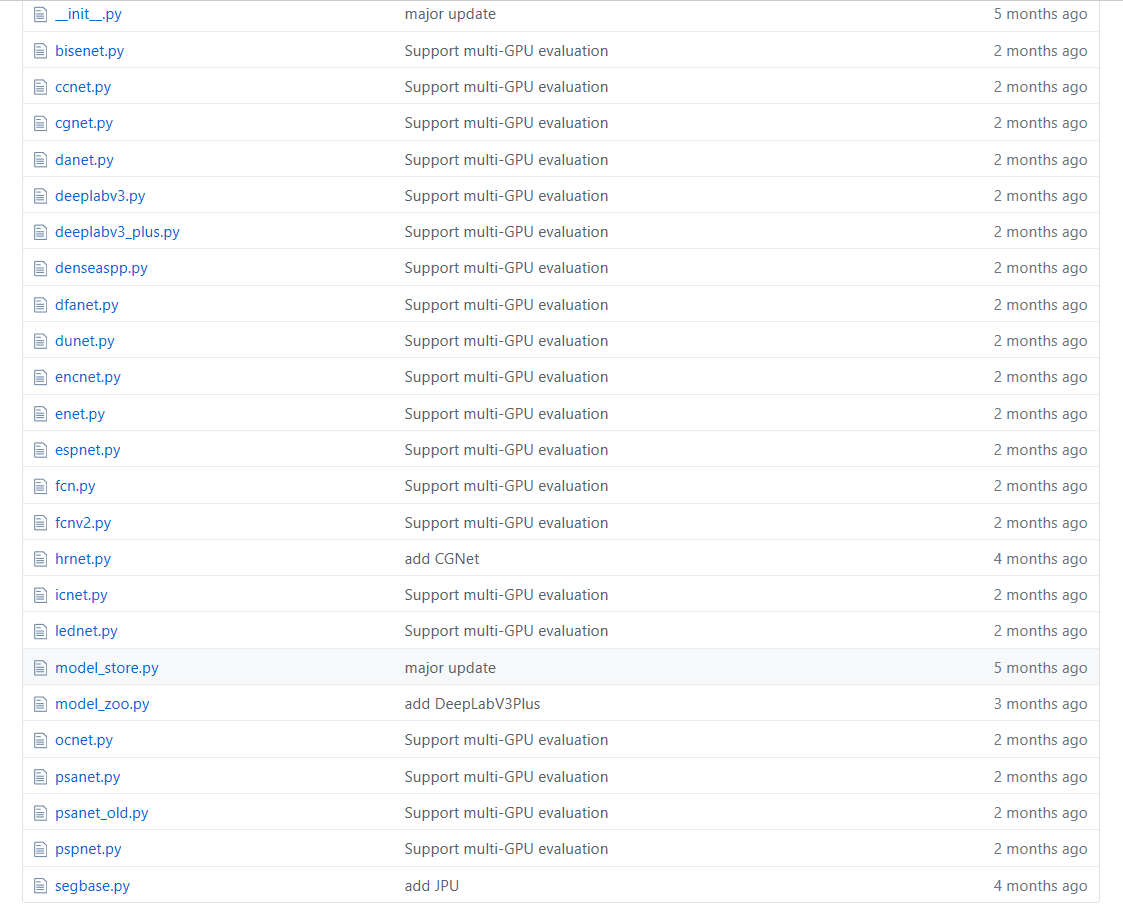展开全文场景分类 语义分割
• 本文是对一些利用了深度学习方法进行SAR影像变化检测的论文的解读，下列是所解读的论文的名称、论文地址和代码地址： 1. Automatic Change Detection in Synthetic Aperture Radar Images Based on PCANet, IEEE ...
本文是对一些利用了深度学习方法进行SAR影像变化检测的论文的解读，下列是所解读的论文的名称、论文地址和代码地址：
1. Automatic Change Detection in Synthetic Aperture Radar Images Based on PCANet, IEEE GRSL 2016.

http://fenggao-file.stor.sinaapp.com/2016_CD_GaborPCANet.pdf
https://github.com/summitgao/SAR_Change_Detection_GarborPCANet

2. Sea Ice Change Detection in SAR Images Based on Convolutional-Wavelet Neural Networks, IEEE GRSL 2019.

http://fenggao-file.stor.sinaapp.com/2019_CD_CWNN.pdf
https://github.com/summitgao/SAR_Change_Detection_CWNN

3. Transferred Deep Learning for Sea Ice Change Detection From Synthetic-Aperture Radar Images, IEEE GRSL 2019.

http://fenggao-file.stor.sinaapp.com/2019_CD_transfered_deep_learning.pdf
https://github.com/summitgao/SAR-Change-Detection-MLFN

4. Change Detection From Synthetic Aperture Radar Images Based on Channel Weighting Based Deep Cascade Network, IEEE JSTARS 2019.

https://ieeexplore.ieee.org/stamp/stamp.jsp?tp=&arnumber=8911214
https://github.com/summitgao/SAR_CD_DCNet

5. SAR Image Change Detection Method via a Pyramid Pooling Convolutional Neural Network, IEEE IGARSS 2020.

https://ieeexplore.ieee.org/stamp/stamp.jsp?tp=&arnumber=9324109
https://github.com/LilWingXYZ/SAR-Image-Change-Detection/blob/main/PSPNet.ipynb（网络复现）

6. SAR Image Change Detection Based on Multiscale Capsule Network, IEEE GRSL 2020.

https://ieeexplore.ieee.org/stamp/stamp.jsp?tp=&arnumber=9036876
https://github.com/summitgao/SAR_CD_MS_CapsNet

7. Bipartite Differential Neural Network for Unsupervised Image Change Detection, IEEE T-NNLS 2020.

https://ieeexplore.ieee.org/stamp/stamp.jsp?tp=&arnumber=8716679

8. Group Self-Paced Learning With a Time-Varying Regularizer for Unsupervised Change Detection, IEEE TGRS 2020.

https://ieeexplore.ieee.org/stamp/stamp.jsp?tp=&arnumber=8915716

9. Unsupervised Scale-Driven Change Detection With Deep Spatial–Spectral Features for VHR Images, IEEE TGRS 2020.

https://ieeexplore.ieee.org/stamp/stamp.jsp?tp=&arnumber=8985538

一、基于 PCANet 的SAR图像自动变化检测（GaborPCANet）
本文提出了一种基于PCANet的SAR图像变化检测方法。如图1所示：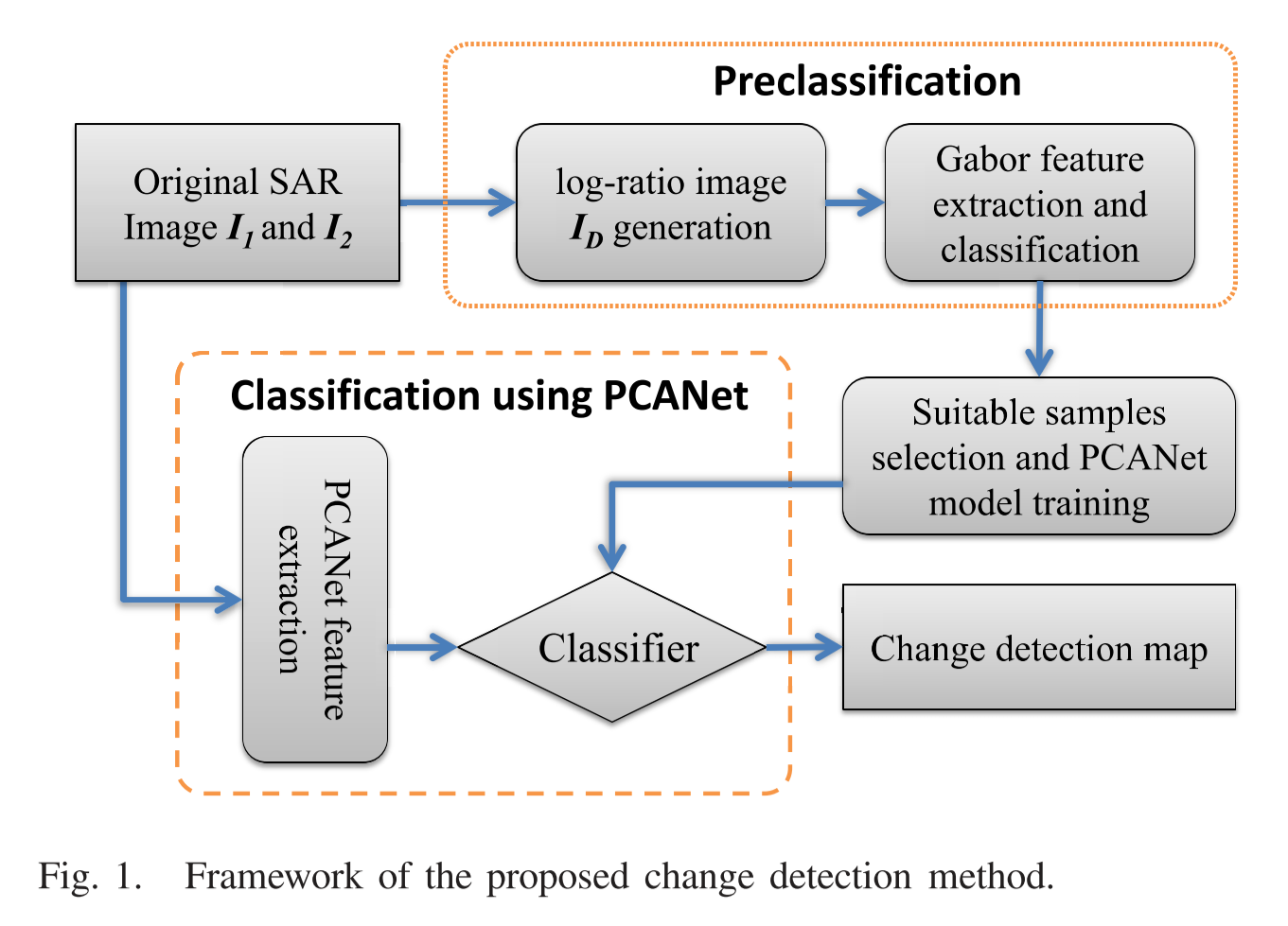1. 预分类：首先利用 log-ratio 算子生成差分图像，然后利用Gabor小波和模糊c均值（FCM）将差分图像中每一个像素分类为变化、不变和不确定三个类别。
2. 将预分类好的变化和不变的两类像素作为训练样本训练PCANet模型。
3. 利用训练好的PCANet模型将第一步中不确定的像素分类，分为变化和不变两类。
4. 将第一步和第三步分类出来的像素结合，形成最终的变化图。

解决的问题及使用的方法：
1.SAR图像存在斑点噪声：
(1) 使用log-ratio算子生成差分图像，能减少斑点噪声的影响。（考虑到SAR图像中斑点的乘法性质，log-ratio算子可以减少斑点噪声的影响。 因此，可以在某种程度上抑制斑点噪声）
(2) 采用PCANet作为变化检测的分类模型，使用PCA滤波器作为卷积核，因为PCANet能够从多时SAR图像中学习非线性关系，所以对散斑噪声有较强的鲁棒性，并能生成噪声较少的变化图。
2.预分类时能分出来确定是变化类和不变类的像素较少，不确定的像素较多，所以难以给PCANet提供足够的训练样本：
(1) 设计了一种预分类方案，使用Gabor小波和FCM为PCANet获得一些高精度的带标记样本。

主要贡献：
1.采用PCANet作为变化检测的分类模型，使用PCA滤波器作为卷积核。
2.设计了一种预分类方案，使用Gabor小波和FCM为PCANet获得一些高精度的带标记样本。

相关技术细节：
Ⅰ.使用Gabor小波和FCM进行预分类
1.通过将差分图像与一组Gabor内核进行卷积来获取差分图像的Gabor小波表示。
Ⅱ.使用PCANet进行分类
1.如图2所示，在图像I1和图像I2上对应的变化和不变的像素点（坐标为(m,n)）周围提取一个大小为 k x k 的 patch，将两个图的patch串联起来，得到大小为2k x k的patch块，这样的一个patch块作为我们的一个样本，我们随机选择N个样本作为我们的训练样本。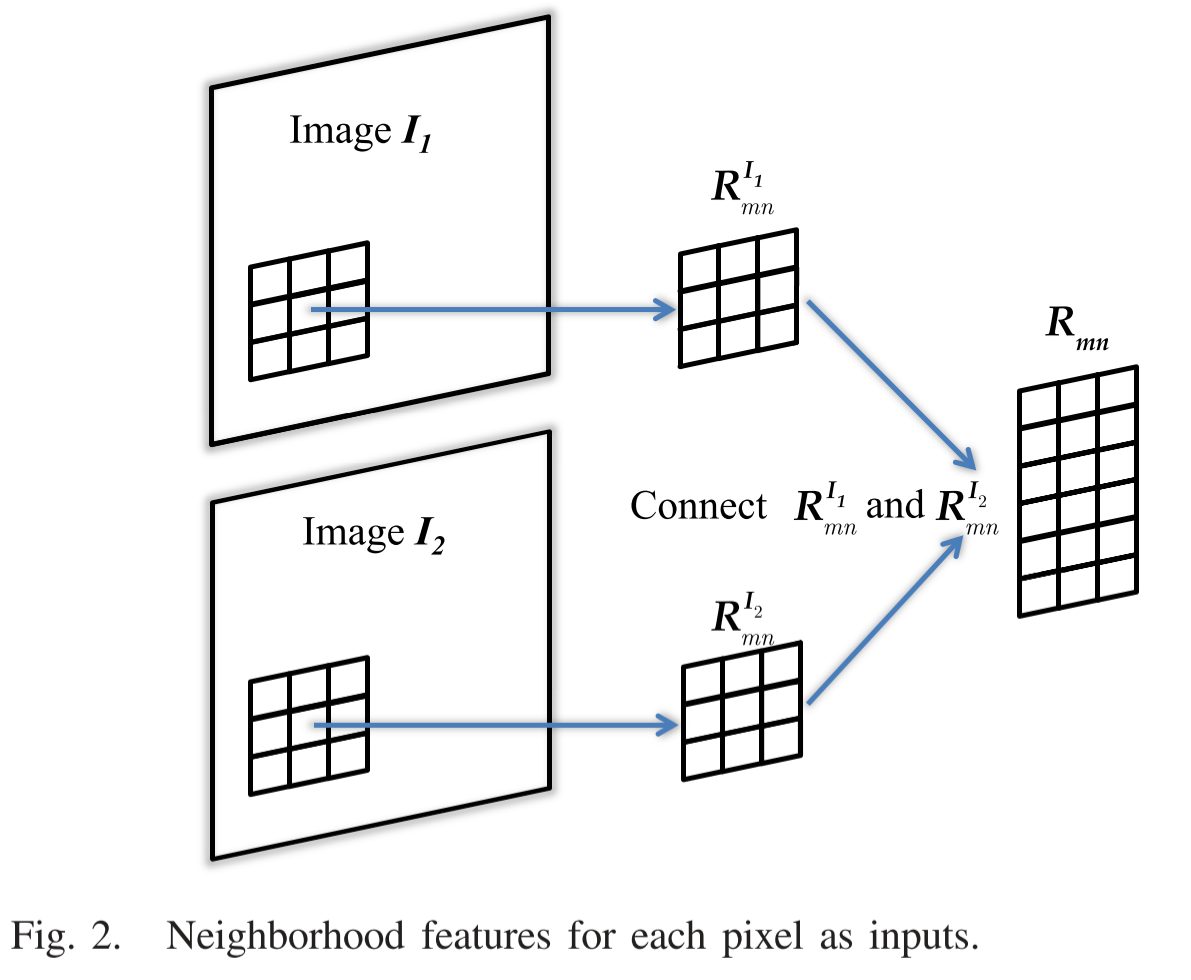2.PCANet的结构如图3所示，它由三个步骤组成：前两个阶段是PCA滤波器卷积，最后一个阶段是散列和直方图生成。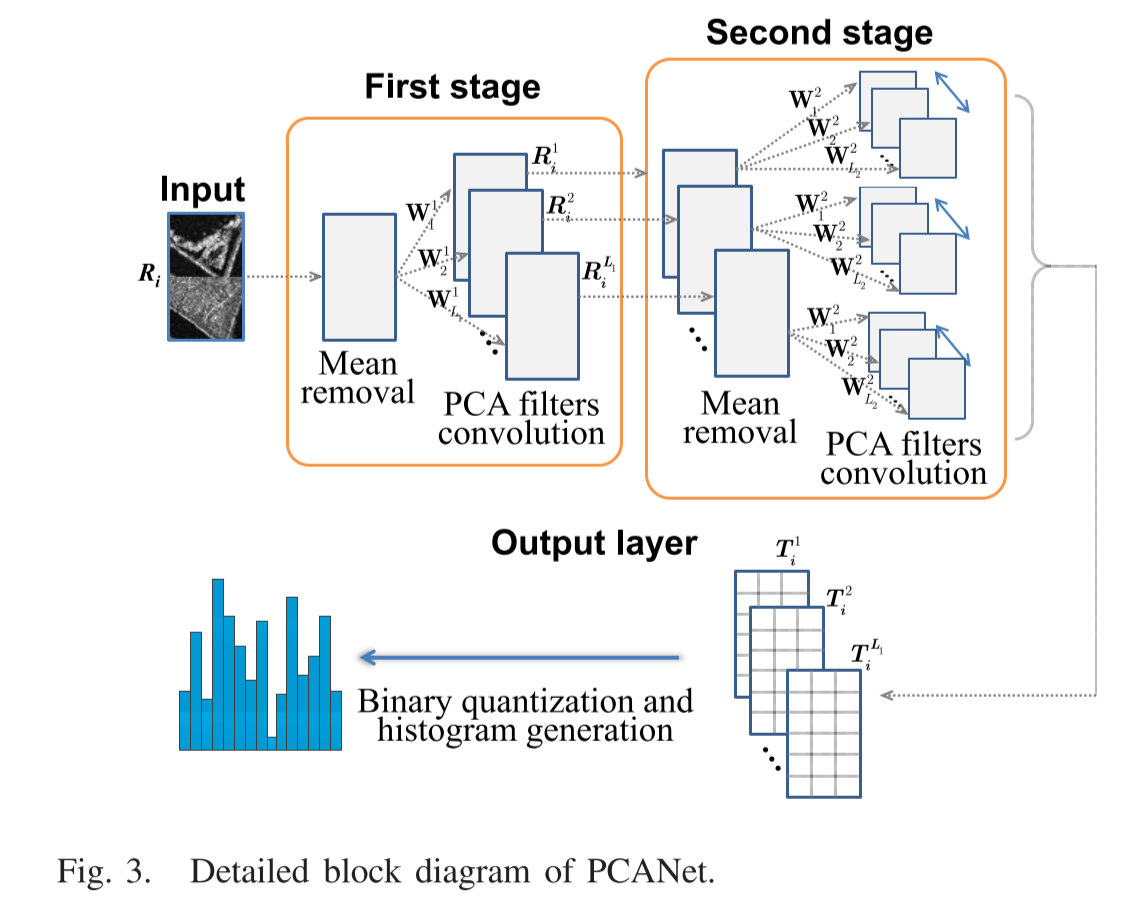二、基于小波卷积的海冰SAR图像变化检测（CWNN）
本文提出了一种基于小波卷积神经网络的海冰SAR图像变化检测方法。如图1所示：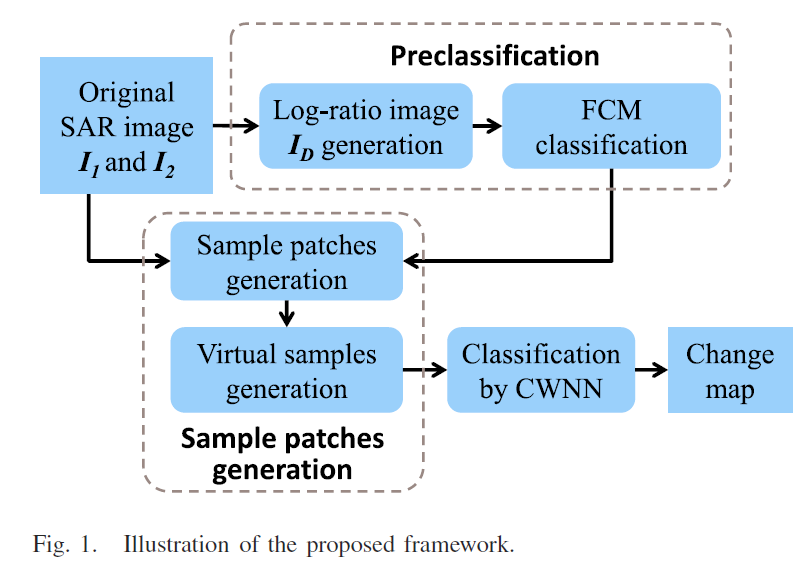1. 预分类：首先利用log-ratio 算子生成差分图像，然后利用模糊c均值（FCM）将差分图像中每一个像素分类为变化、不变和不确定三个类别。
2. patch样本生成：生成每个像素周围的patch样本，并且还生成一些虚拟patch样本，将预分类好的变化和不变的两类patch作为训练样本。
3. 通过CWNN分类：利用第2步的训练样本训练CWNN模型，再用训练好的CWNN模型将原始patch全部分类，形成最终变化图。

解决的问题及使用的方法：（主要贡献）
1. SAR图像存在斑点噪声：
（1）提出基于小波卷积的变化检测方法：将双树复小波变换（DT-CWT）引入到卷积神经网络中，对变化的像素和不变的像素进行分类，从而有效降低了斑点噪声的影响。
2. 训练样本有限：
（1）采用虚拟样本生成方案来创建用于CWNN训练的样本，从而减轻了训练样本有限的问题。

相关技术细节：
Ⅰ. Patch样本的生成
1. 在原始SAR图像I1和图像I2上对应的变化和不变的像素点周围提取一个大小为 w x w 的 patch，将两个图的patch串联起来，得到大小为2w x w的patch块，这样的一个patch块作为我们的一个样本，总共选择N个训练样本（N为所有变化和不变的patch的总和）。
2. 由两个相同类别的样本以适当的比率产生一个虚拟样本，所以虚拟样本的类别和这两个样本保持一致，公式如下：Pk = αPi + (1-α)Pj + β，其中Pi和Pj是来自同一个类的两个训练样本，α是区间[0，1]上均匀分布的随机值，β表示随机高斯噪声，β的均值设为0，方差设为0.001。最后将真实样本和虚拟样本一起用作训练样本。
Ⅱ. 小波卷积神经网络分类
1. CWNN框架图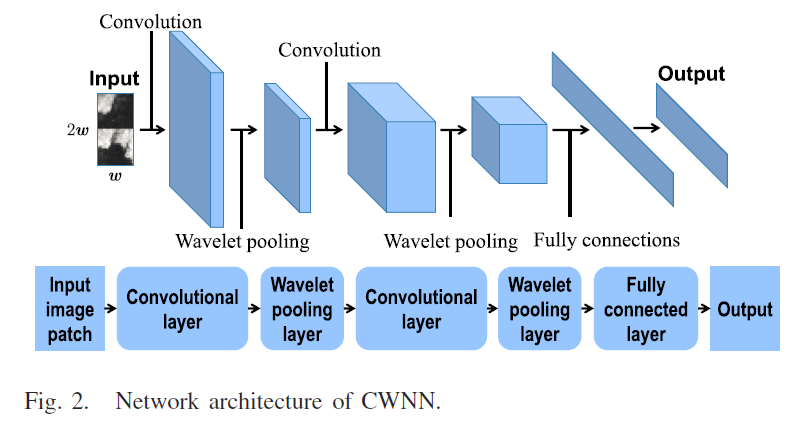CWNN的结构如图2所示。两个卷积层表示为C2和C4，一个小波池化层表示为W3和W5。因此，网络可以表示为{I1、C2、W3、C4、W5、F6、O7}。i1是输入层，所有输入图像块都被重采样为28 × 14的大小。C2是一个具有6个卷积核的卷积层，其大小为5×3。该层生成六个大小为24 × 12的feature map。W3是一个小波池化层，在该层中，所有的输入特征图都用一级DT-CWT分解，该小波池化层产生6个大小为12×6的特征图。C4是具有12个卷积核的卷积层，其大小为5×3，该层生成12个8×4大小的feature map。w5是一个小波池化层，它产生12个大小为4×2的特征图。F6是具有96个单元的全连接层。o7是带有两个单元的输出层，这两个单位分别代表变化和未变化的类。最后，我们将CWNN分类结果和预分类结果结合在一起，形成最终的变化图。
2. 小波池化层
池化层之前的层可以通过双树复小波变换（DT-CWT）分解成八个组成部分。八个分量包括两个低频子带L1和L2以及六个方向的高频子带，正负15°，正负45°，和正负 75°(由LH1、LH2、HL1、HL2、HH1和HH2表示)。选择两个低频子带的平均值作为池化层的输出。

三、基于迁移学习的海冰SAR图像变化检测（MLFN）
本文提出了一种迁移多级融合网络（MLFN），用于海冰SAR图像变化检测。如图1所示：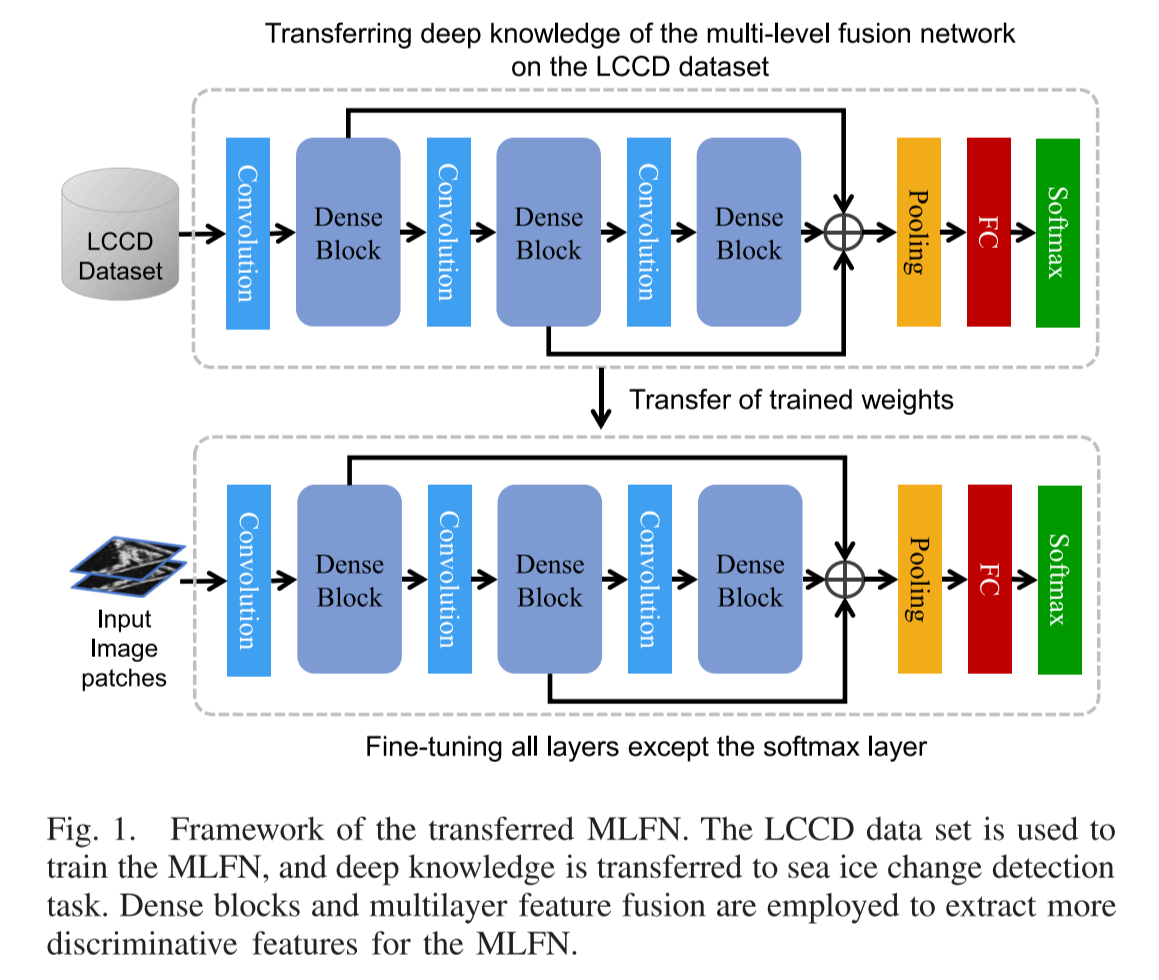1. 首先使用一个大型数据集（LCCD）来训练MLFN：从LCCD数据集中随机抽取大量patch对，再将这些patch对送到MLFN中用作训练样本。
（1）用级联Dense Block来优化卷积层： 使用三个Dense Block进行特征提取，分别提取低级，中级和高级特征。（引入了多层特征融合以利用低，中和高级特征表示之间的互补信息， 因此，MLFN可以实现更具区分性的特征提取）
2. 训练后，将训练好的权重迁移到海冰SAR图像变化检测任务当中。
（1）预分类：首先利用log-ratio 算子生成差分图像，然后利用Gabor小波和模糊c均值（FCM）将差分图像中每一个像素分类为变化、不变和不确定三个类别。
（2）微调：将预分类好的变化和不变的两类像素用于微调MLFN模型，优化MLFN的网络参数。（同样采用抽取patch的方式）
（3）利用微调好的MLFN模型将(1)中不确定的像素分类，分为变化和不变两类。
（4）将(1)和(3)中分类出来的像素结合，形成最终的变化图。

解决的问题及使用的方法：
1.海冰数据集较少，在变化检测任务中具有局限性：
（1）使用一个大型数据集（LCCD）来训练MLFN，然后将丰富的知识迁移到海冰分析中。（因为CNN较低层的特征对分类任务的针对性较小）

相关技术细节：
Ⅰ.多层融合网络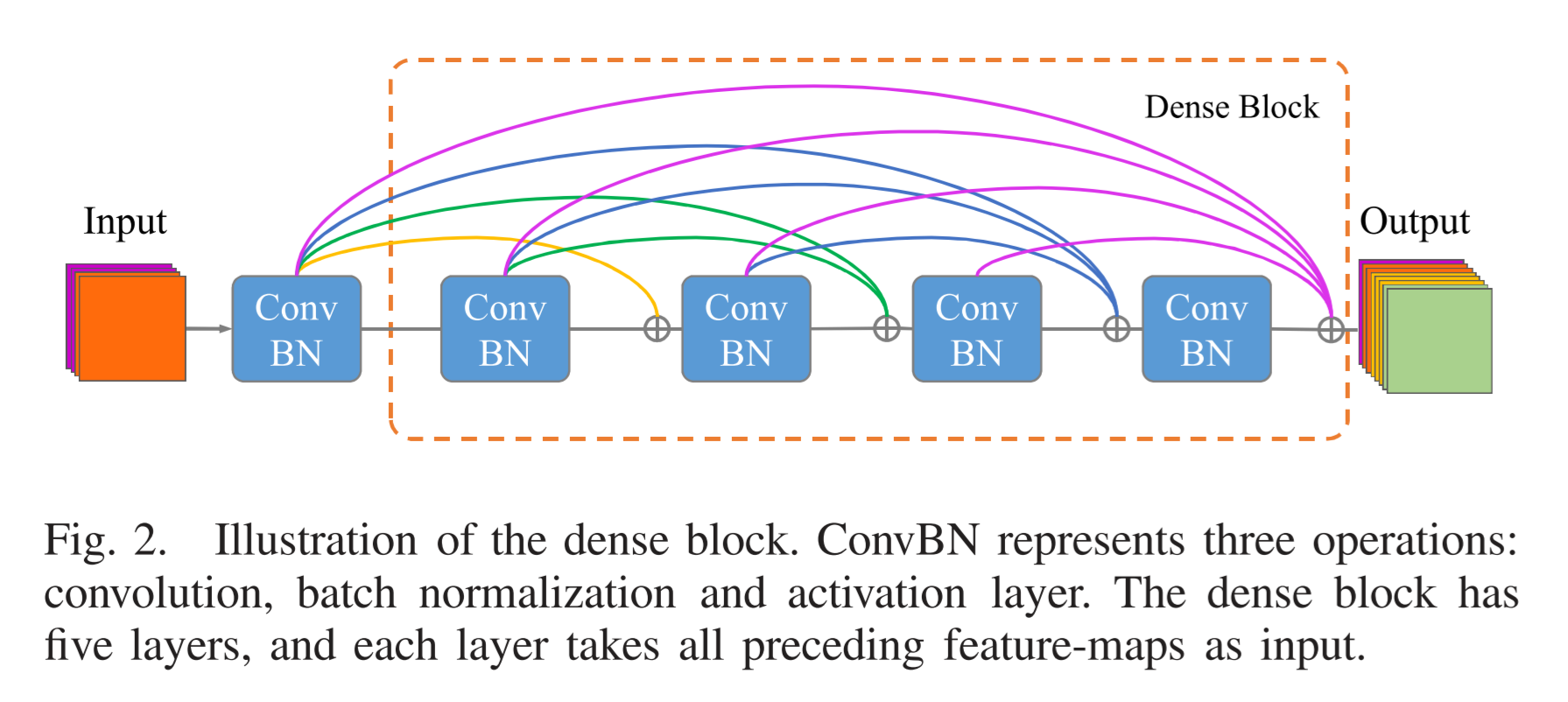DenseNets是残差网络的极端情况，其中每个卷积层都通过多个快捷连接进行连接，加强了特征传播并鼓励了特征重用，在一定程度上减少参数数量。图2是Dense Block的内部框架结构，MLFN使用了三个Dense Block进行特征提取，第一个Dense Block可以提取图像的次要细节，例如线条或点；第二个Dense Block可以提取中级特征，这些特征对应于低级特征的组合输出；最后，第三个Dense Block可以捕获输入图像的结构化信息和语义上下文。
如图2所示，Dense Block具有四层。 第l层具有l个输入，由所有先前卷积层的特征图组成。 它自己的特征图将传递到所有后续层，这在Dense Block中引入了4×（4 +1）/ 2 = 10个连接。
在图1中，已经介绍了MLFN所采用的特征融合策略是利用三个Dense Block， 这三个模块的输出分别表示为FL，FM和FH。 这三个块的输出合并结果为：F=pooling（h(FL) + h(FM) +h(FH)），其中F表示融合特征，池化是全局平均函数，h是尺寸匹配函数，在特征融合之前执行尺寸匹配。，使用大小为1×1的64个内核对FL，FM和FH进行卷积。 通过这种卷积，三个Dense Block的特征图的数量全部变为64。因此，可以通过逐元素求和来实现特征融合，融合后送到全局池化层，然后由一个全连接层进行处理。 最后，将融合特征转换为一个高维向量用作softmax图层的输入，以计算变化或不变的可能性。 可以将变化或不变的可能性分别表示为pc和pu。 如果pc> pu，则输入图像的patch对属于变化类别，否则属于未变化类别。
Ⅱ.微调
有两种可能的方法可以在预训练的MLFN中执行微调。第一种方法是微调所有图层。第二个是保持一些低层固定，然后微调高层。在第一种方法中，从预训练的MLFN中移除softmax层。在另一种方法中，冻结低层以保持已经学习的特征，并针对输入的多时间SAR图像调整高层。在这篇文章中通过实验表明微调所有层的特征对于海冰图像变化检测结果有所提高。

四、基于通道加权的深度级联网络的SAR图像变化检测（DCNet）
本文提出了一种用于SAR图像变化检测的深度级联网络（DCNet）。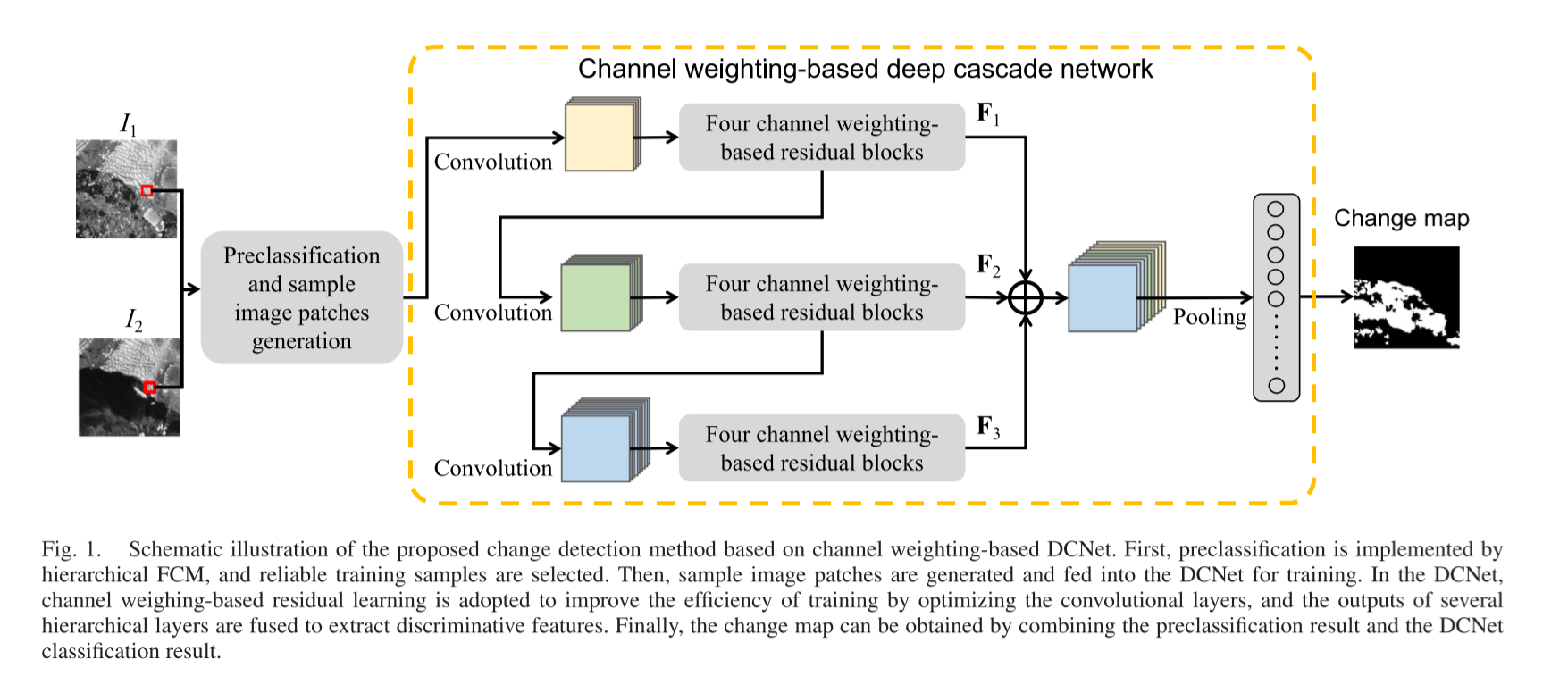1. 预分类：首先利用log-ratio 算子生成差分图像，然后利用分层聚类算法对差分图像进行分类，在分层聚类算法中，FCM算法用于将差分图像中每一个像素分类为变化、不变和不确定三个类别。
2. 将预分类好的变化和不变的两类像素作为训练样本训练DCNet模型。
（1）引入残差学习优化卷积层
（2）基于通道加权的残差块可以利用特征通道的关系
（3）将不同层的输出融合以形成最终特征集
3. 利用训练好的DCNet模型将第一步中不确定的像素分类，分为变化和不变两类。
4. 将第一步和第三步分类出来的像素结合，形成最终的变化图。

解决的问题及使用的方法：（主要贡献）
1. 随着网络深度的增加，卷积神经网络经常会遇到一些负面影响，例如过拟合和梯度爆炸。
（1）引入残差学习来解决梯度爆炸问题。
（2）引入特征融合机制来组合不同层的输出，以进一步缓解梯度爆炸问题。
2. SAR图像变化检测中使用的现有深层网络往往会产生许多冗余特征（每个卷积运算都会生成一组特征图），这些不必要的特征会影响网络的性能。
（1）设计了一个基于通道加权的模块，平均池和最大池用于汇总通道信息，强调有意义的通道，抑制不必要的通道。因此，可以降低特征图的相似度，减少冗余特征，进而提高DCNet的分类性能。

相关技术细节：
I. 预分类和样本选择
1.如图2所示，从两幅原始SAR图像的潜在变化区域中提取以所选样本为中心的patch块（两幅图像所选取的位置为同一位置），每个patch的大小为r×r，将两个patch组合在一起以形成具有两个通道的新图像Rk，Rk的大小为r×r×2。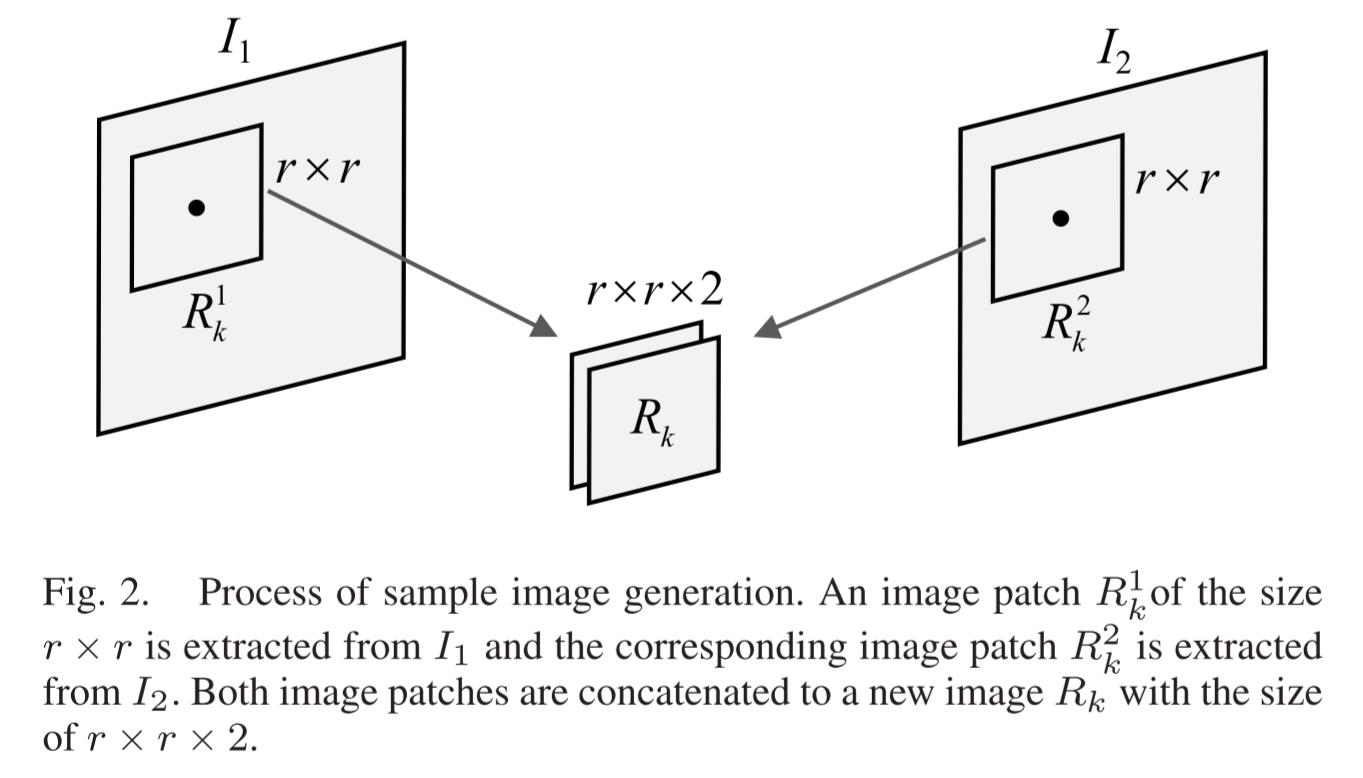Ⅱ. 深度级联网络（DCNet）
DCNet包含两个重要组件：基于通道加权的残差块和特征融合模块。
1. 基于通道加权的残差块：由于残差图像的大多数值很可能为零或趋于较小，因此残差网络更易于训练。 一个典型的残差块如图3所示，它通过块内的快捷路径直接连接输入和输出。DCNet中设计了一个基于信道加权的残差块，如图4所示。DCNet通过具有不同参数的基于级联信道加权的残差块来实现更深的网络深度，级联的残差块可以完全提取SAR图像的特征信息。如图1所示，在DCNet中有三组基于信道加权的残差块。它们分别用于提取低，中和高级特征。 第一组残差块可以提取图像的次要细节，例如线或点；第二组残差块可以提取与低级特征的组合输出相对应的中级特征；第三组残差块可以捕获输入数据的结构化信息和语义上下文。 每个组由四个具有相同参数的基于信道加权的残差块组成。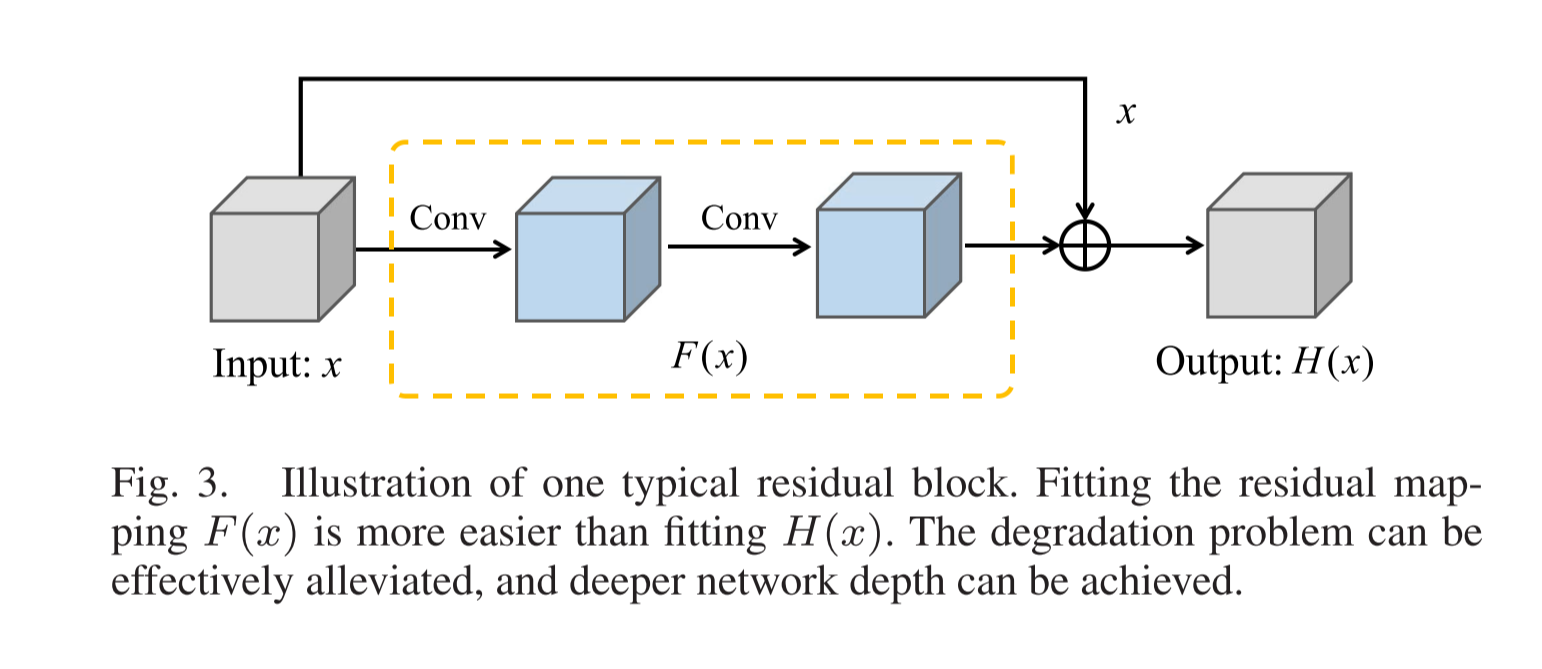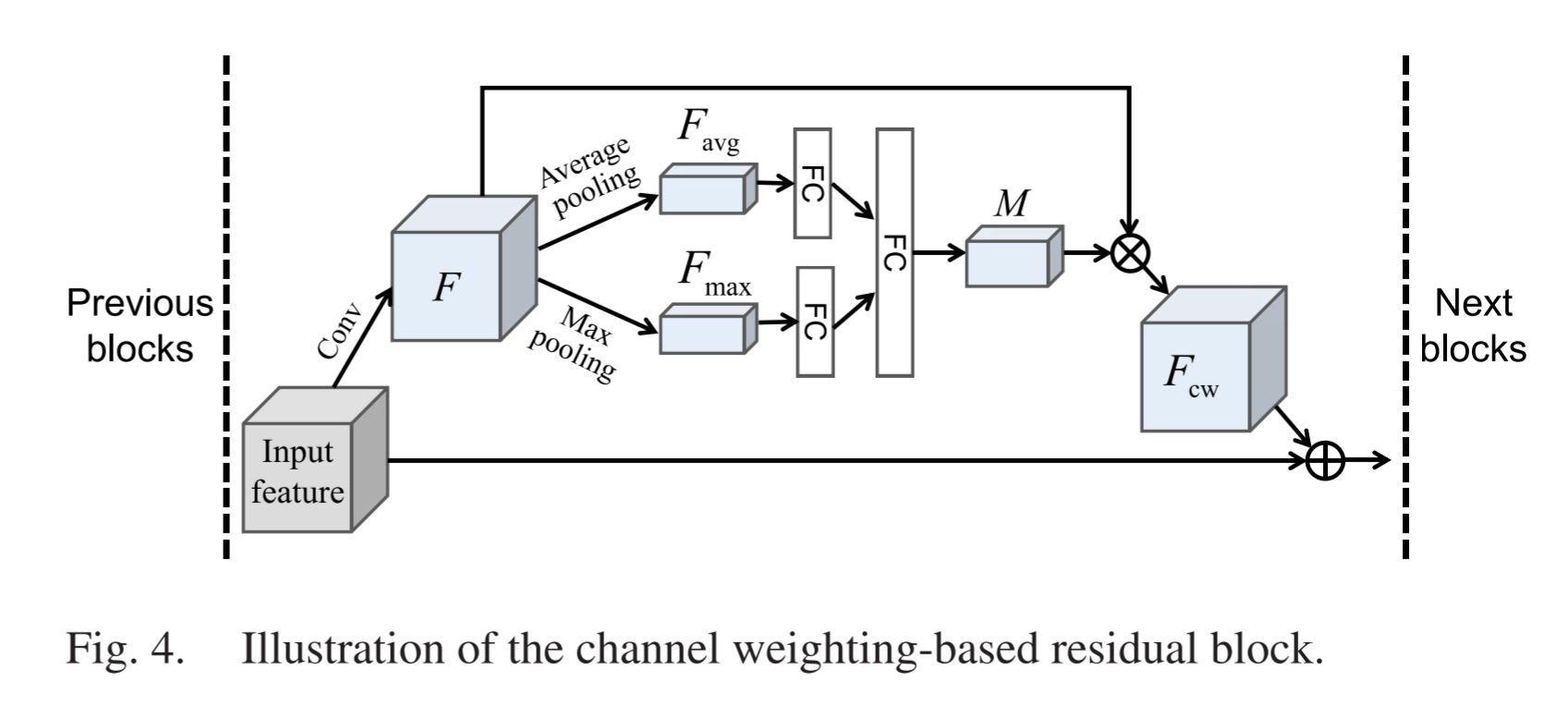2.特征融合： 该文章引入了特征融合策略来研究三组基于通道权重的残差块中的互补信息。特征融合策略的工作方式与残差学习类似，具体来说，残差学习结合了相同尺度的特征，而特征融合策略则结合了不同尺度的特征。因此，特征融合策略可以看作是残差学习的扩展版本，从而可以进一步缓解爆炸梯度问题。
不同级别的级联残差块可以捕获不同尺度的信息，包括粗糙或精细尺度，因此级联残差块的不同级别特征的融合对于变化检测非常重要。 将三个基于信道加权的残差块的特征分别表示为F1，F2和F3，F1包含16个特征图，F2包含32个特征图，F3包含64个特征图。 为了在特征融合时保持尺寸一致，使用64个 kernels 对F1，F2和F3进行卷积，每个 kernel 的大小为1×1，经过这种卷积，三组输出的总数全部变为64。之后，可以通过逐像素求和来实现融合过程，公式如下：F = g1(F1)+g2(F2)+g3(F3)， 其中F表示融合特征，g1，g2和g3是尺寸匹配操作。

六、基于多尺度胶囊网络的SAR图像变化检测（Ms-CapsNet）
本文提出了一种用于SAR图像变化检测的多尺度胶囊网络（Ms-CapsNet）。如图1所示：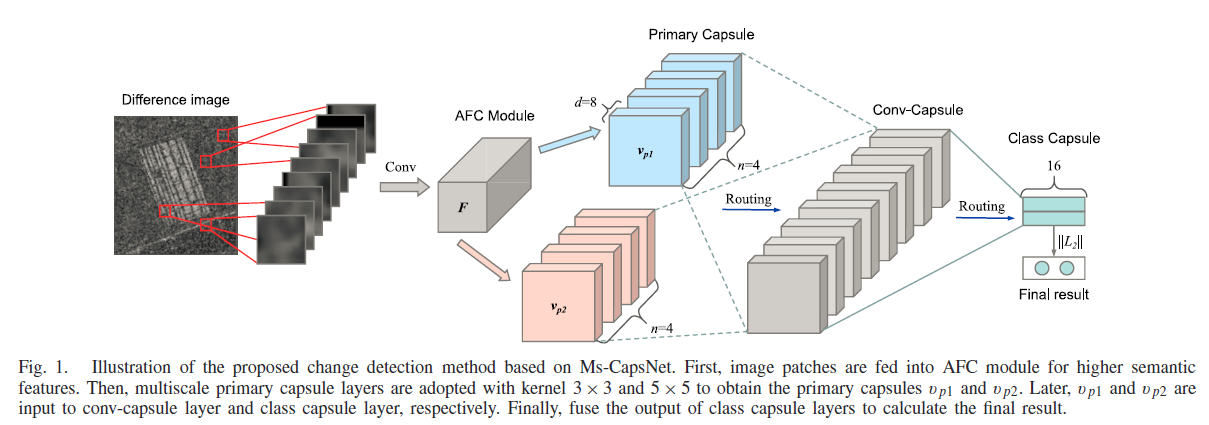1. 首先利用 log-ratio 算子生成差分图像。
2. 然后从差分图像中随机选择训练样本用于Ms-CapsNet训练。
（1）Ms-CapsNet 由自适应融合卷积模块（AFC）和胶囊模块组成
（2）AFC模块用于将像素强度转换为高语义特征
（3）胶囊模块用于激活高语义特征
3. 最后利用训练好的Ms-CapsNet对差分图像中的像素进行分类，得到最终的变化图。

解决的问题及使用的方法：（主要贡献）
1. 不同位置特征的相关性不能有效建模：
所提出的 Ms-CapsNet 具有从不同位置提取鲁棒特征的能力，它提供了一组实例化参数来从不同的位置捕获特征，因此可对不同位置特征的相关性建模，从而也解决了训练样本不够的问题。
2. SAR影像中固有的散斑噪声：
设计了一个自适应融合卷积模块（AFC），可以有效地将像素强度转化为局部特征的活动。AFC模块通过注意力策略提取较高的语义特征并强调有意义的特征，因此局部特征的活动变得更具噪声鲁棒性。

相关技术细节：
Ⅰ. AFC模块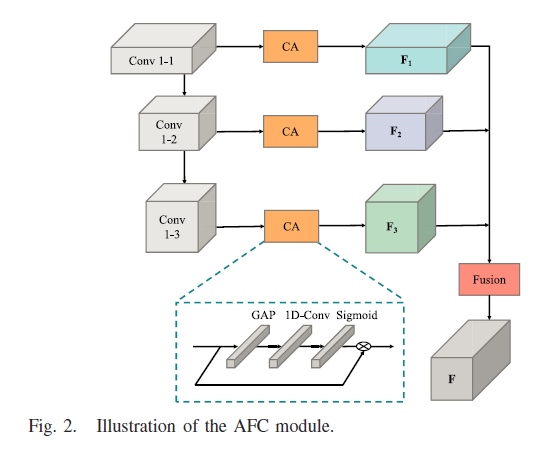如图所示，利用AFC模块对输入进行编码。
首先，一组卷积（Conv1-1、Conv1-2和Conv1-3，其核大小为3×3）采用了不同的空洞率（分别设置为1、2、3），以捕获多尺度特征，得到输入特征 Fin （w0w0c0）。
然后将输入特征 Fin 输入到通道注意力模块（CA）中，利用全局平均池化（GAP）在空间域挤压 Fin 得到 Favg （11c0），然后利用一维卷积来找 Favg 中的通道关系，然后通过Sigmoid函数得到一个基于通道加权的向量 M，最后，将 Fin 和 M 结合起来得到基于通道加权的特征 Fout（Fout=M⊗Fin，其中⊗表示通道乘法）。来自Conv1-1、Conv1-2和Conv1-3的基于通道加权的特征分别表示为F1、F2和F3。
最后对多尺度特征进行特征融合，通过逐像素求和法将特征融合为：F = D1(F1) + D2(F2) + D3(F3)，其中F表示融合特征，D1、D2和D3是利用1×1卷积实现的维数匹配函数。

Ⅱ. 胶囊模块
胶囊模块是由初级胶囊层、Conv胶囊层和类胶囊层组成的神经网络，如图1所示。
1. Primary Capsule Layer
该层通过核大小为k×k的类卷积操作从多维实体中提取低层次特征。与传统卷积不同，它将获得多个特征图而不是一个。初级胶囊层首先从AFC模块接收大小为 wwc 的特征图。 然后采用类卷积运算和压缩函数得到输出胶囊 vp。压缩函数表示为：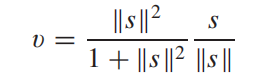其中s是总输入，v是胶囊的矢量输出。 在初级胶囊层中，输出胶囊vp的大小为w1映射，n×w1×n×d，其中n是特征×d=c的数目，d是8。 [w1×w1]网格是共享的权重。 换句话说，我们在总初级胶囊中获得[w1×w1×n]8D载体。 在我们的实现中，考虑了多尺度信息。 两个初级胶囊层分别采用核大小k=3和k=5。 因此，可以得到多尺度特征表示。 来自两个尺度的特征向量分别用vp1和vp2表示。
2. Conv-Capsule Layer
该层采用局部连接和共享变换矩阵，在一定程度上减少了参数的。 卷积胶囊层采用动态路由策略更新耦合系数c。原囊层与conv-囊层之间的连接（变换矩阵）为W，变换矩阵W也每个网格中也共享。 因此，conv-胶囊层的输出vc可以表示为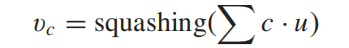其中c是耦合系数，u=W·vp.vp是初级胶囊层的输出。 对于动态路由，我们首先将协议b设置为0。 耦合系数c可以用c=softmax(b)计算)。 也就是说，我们更新b来计算最新的耦合系数c.此外，b的更新过程可以表示为b←bu·vc。
3. Class Capsule Layer
类胶囊层可视为完全连接层。动态路由机制仍用于耦合系数更新。 在这一层中，通过求和VO1∈VO2×VO2融合类胶囊层中的多尺度活动向量VO1⊕R2和VO2。 然后，计算向量范数来度量类的概率。 可以将ms-Caps Net的损失函数定义为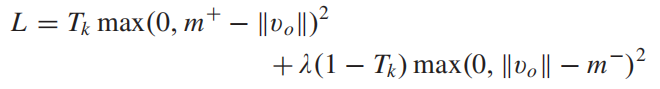当标签k出现时，tk=1(k=0表示不变的类，k=1表示更改的类)。 λ=0.5用于约束初始类胶囊的活动向量的长度。 如果图像中有一个更改类对象，则更改类的类包应该输出一个长度至少为m=0.9的向量。 相反，如果没有改变类的对象，则从类胶囊中输出长度小于m−=0.1的向量。 然后，通过像素分类可以计算出最终的变化图。


展开全文SAR图像
• 写在前面 本篇文章主要总结梳理了以下两篇综述的内容...在城市规划，环境监测，农业调查，灾害评估和地图修订中，变化检测技术具有广泛的应用。由气候变化引起的极端灾害的数量不断增加，例如干旱，洪水，飓风和热浪，计算机视觉 人工智能
• 深度学习物体检测常用资源综述，包括R-CNN 、Fast-RCNN、Faster-RCNN、YOLO、SSD等
• 参考：https://blog.csdn.net/Standing_On_Giant/article/details/60333329参考：...目前业界出现的目标检测算法有以下几种：1. 传统的目标检测算法：Cascade + Haar / SV...
• 首先我们应该我们了解到遥感图像的变化检测是建立在多时相的基础上的，也就是说，我们对于神经网络的输入至少是有两张图片的，而且必须有一个标签来知道变化的区域的是那一块。 在双时相变化检测里，一般来说对于...机器学习 人工智能 tensorflow python
• 基于深度学习的机器视觉外观检测软件 以前，我们不可能通过深度学习技术来执行自动化检测任务。如今，越来越多的制造商正在转向基于深度学习的图像分析软件来帮助提升许多设备的自动化执行水平。 软件可以容忍偏差...机器视觉 工业4.0 人工智能
• 本篇博客主要转载两篇写得好的分别介绍基于深度学习的目标检测和人脸检测的文章，最近在调研基于深度学习的人脸检测相关的文章，在网上查相关资料时，有幸看到。文末附带基于深度学习的目标检测和人脸检测相关经典...
• 目标检测是图像处理和计算机视觉学科的重要分支，对人脸识别、步态识别、人群计数、实例分割等任务起着至关重要的作用。 计算机视觉对于目标运动的分析可以大致分为三个层次: 运动分割，目标检测 --&amp;gt; ...
• 仍然有许多应用需要最先进的解决方案，例如，强烈的背景变化或不断变化的光照。蓝色工具将完成非常具有挑战性的OCR任务。第一步该工具将学习找到和定位图像中的字符，忽略它们本身的意思。一旦可以可靠地运作，蓝色...机器视觉
• ”谈谈深度学习目标检测中的遮挡问题 - Naiyan Wang的文章 - 知乎 https://zhuanlan.zhihu.com/p/43655912   今天给大家介绍的两篇工作的目的都是试图解决目标检测中最为棘手的遮挡问题。两篇文章的作者分别从...
• 深度学习中的目标检测：概述 本文由CVPy翻译自以下文章： https://tryolabs.com/blog/2017/08/30/object-detection-an-overview-in-the-age-of-deep-learning/。 前言 从简单的图像分类到3D姿势估计，计算机视觉...计算机视觉
• （自己写的，需要转载请联系作者，或者标明出处呀，欢迎加微信交流：wx604954） ...本文介绍了目前发展迅猛的深度学习方法在目标检测中的最新应用进展，然后介绍了基于深度学习的目标检测算法在医学图像领域的应...
• 深度学习目标检测经典模型比较（RCNN、Fast RCNN、Faster RCNN）   Faster rcnn是用来解决计算机视觉(CV)领域中目标检测(Object Detection)的问题的。 区别目标分类、定位、检测 一、传统的目标检测方法 其实...RCNN Fast RCNN
• ## 深度学习车道线检测

千次阅读 多人点赞 2020-06-20 05:44:52
深度学习检测车道线(一) 2019-01-19 10:37:41qq_27546529阅读数9750 中级课 Opencv4图像分割和识别实战课程-车道线检测相关理论知识点-计算机... 第九章:车道线检测1.车道线检测相关理论知识点 [ 27:19 ] ...
• ## 深度学习与图像识别 图像检测

万次阅读 多人点赞 2016-11-25 17:08:40
主要是看了些关于深度学习图像识别和图像检测的论文，介绍了深度学习处理图像的优势图像识别
• 红色工具都能通过学习对象的正常外观，包括重要的、允许的变化，识别这些问题乃至其他更多的问题。使用 ALFA 红色工具，您只需要提供拥有正常表象的图片。ALFA红色工具有两种工作模式：• 异常检测器：非监督...人工智能 机器视觉
• 基于深度学习的目标检测算法归类和总结 整体框架 目标检测算法 主要包括：【两阶段】目标检测算法、【多阶段】目标检测算法、【单阶段】目标检测算法 什么是两阶段目标检测算法，与单阶段目标检测有什么区别？ 两...
• ## 基于深度学习的目标检测算法综述

万次阅读 多人点赞 2018-06-19 16:04:53
其它机器学习、深度学习算法的全面系统讲解可以阅读《机器学习-原理、算法与应用》，清华大学出版社，雷明著，由SIGAI公众号作者倾力打造。 书的购买链接 书的勘误，优化，源代码资源 导言 目标检测的任务是找出...机器学习 神经网络
• 一、目标检测任务 1、任务综述 给定一张图像或者视频帧，找出其中所有...图像中的目标可能出现在任何位置，目标的形态可能存在各种各样的变化。 2、模型框架 传统的目标检测中，常见的算法是DPM（Deformable Pa
• 点击上方“3D视觉工坊”，选择“星标”干货第一时间送达作者：Mia Morton编译：ronghuaiyang(新机器视觉）导读创建异常检测模型，实现生产线上异常检测过程的自动化。在选择数...python 计算机视觉 机器学习 人工智能
• 本篇文章是精读了三篇目标检测综述文章之后，结合落地项目经验总结而成。文章内容很多，本文的目的是划重点，写核心，使刚入门的同学对目标检测有从感性到理性的认识。 文章中出现的论文，全部打包整理，需要仔细...
• 国庆假期闲来无事~又正好打算入门基于深度学习的视觉检测领域，就利用这个时间来写一份学习的博文~本博文主要是本人的学习笔记与调研报告（不涉及商业用途），博文的部分来自我团队的几位成员的调研报告（由于隐私...
• 深度学习目标检测网络汇总对比 本文总阅读量次 欢迎star我的博客 2019-01-03 参考 ：...
• 经过深度学习的训练，可以预测新图像上是否有瑕疵和这些瑕疵的位置(以下这张图是未参加训练的测试图)。 实验效果 经过深度学习训练后得出一个模型，利用该模型对测试图片进行预测，其预测效果如下图 实验过程...Darknet YOLOV3
• faster RCNN模型是在fast RCNN的基础上，把候选区域集成到统一的网络中，形成端到端的检测模型。faster RCNN可以简单地看做“区域生成网络 + fast RCNN“的系统，用区域生成网络RPN代替fast RCNN中的Selective ...
• 基于深度学习的目标检测研究进展 目标检测领域得益于卷积神经网络CNN和region proposal算法的发展，在各种大规模竞赛数据集（ImageNet，Pascal VOC,COCO等）上准确率突飞猛增。 目标检测中非常重要的指标：识别...
• ## 基于深度学习的视频检测（一）

万次阅读 多人点赞 2017-09-04 16:47:44
一、简介图像目标检测任务在过去几年深度学习的发展背景下取得了巨大的进展，检测性能得到明显提升。但在视频监控、车辆辅助驾驶等领域，基于视频的目标检测有着更为广泛的需求。由于视频中存在运动模糊，遮挡，形态......